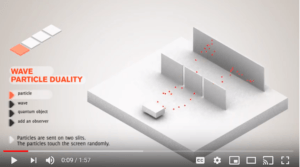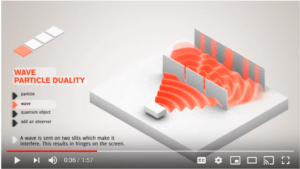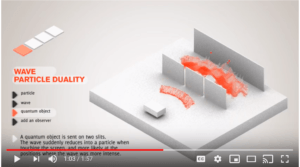Home » Archives for June 2019

# What is the difference between quantum mechanics and quantum physics?

Both “quantum mechanics” and “quantum physics” mean the study of subatomic particles. But “quantum mechanics” is more specific. It’s the term used for the field once it was formulated into mathematical laws. Then, it became a kind of mechanics. Prior to the development of mathematical laws governing subatomic particles, the field was called “quantum theory” or “quantum physics.” The distinction between “quantum mechanics” and “quantum physics” is observed by physicists but not necessarily by lay people.

## More detail on the difference between quantum mechanics and quantum physics

In the early 1900’s, physicists, through experiments, discovered that atoms and their components followed different laws from those of ordinary objects like tables and chairs. The mathematical laws governing the movements and forces among ordinary objects is known as “classical mechanics” or “Newtonian mechanics.” For example, Force = Mass times Acceleration is a mathematical law of classical mechanics.Drawing of a photon (in green) being emitted from carbon molecules. [Image source: Nancy Ambrosiano, Los Alamos National Laboratory, July 2017 News Release, “Single-photon emitter has promise for quantum info-processing,” (Public domain)]

When physicists realized that quantum particles do not follow the laws of classical mechanics, they called the new field “quantum theory.” At first, physicists developed quantum laws which were heavily verbal, rather than highly mathematical.

In the 1920’s, physicists developed mathematical laws which describe quantum behavior. In particular, Erwin Schrodinger and Werner Heisenberg developed the key mathematical laws governing quantum particles (Schrodinger’s Wave Equation and Heisenberg Matrix Mechanics). At this point, physicists began calling the new field “quantum mechanics” on the model of the phrase “classical mechanics.”

The term “quantum mechanics” means the same thing as “quantum physics” though the term “mechanics” emphasizes doing calculations.

# What is wave function collapse? Is it a physical event?

In one view, a wave function is a piece of math, an equation. It’s not a physical thing. So, it can’t collapse in any physical sense. The collapse is metaphorical. This is one interpretation of quantum mechanics. It’s the interpretation taught in most university classes, the Copenhagen Interpretation. However, physicists have not settled on a particular interpretation. For more nuance, see the later section “Caveat—Other Interpretations of Quantum Mechanics.”

To continue on with the view that the wave function is a piece of math: a wave function is, first of all, a function—just like the functions in algebra—a very common type of equation. The reason that physicists call it a “wave function” is that it’s an equation that when graphed, looks like waves. The accompanying  image shows a graph of a particular wave function at a particular moment in time. It graphs as waves with six three-dimensional peaks:This wave function could describe an electron in a box, possibly imprisoned by magnetic fields. The graph tells us the likelihood of detecting the electron in any particular position in the box. It gives us a set of probabilities as to the likely position of the electron when detected. We’ll need to square the amplitude (wave height) that we’ve calculated for each position to find out the probability of detecting the electron in that position.

For this graph, the calculation of the wave function tells us that the electron has an equal probability of being detected in six different positions (A, B, C, D, E, or F) and a negligible probability of being detected elsewhere.

## Collapse of the Wave Function

Upon detection of the electron, the probabilities calculated by the wave function instantaneously convert to a 100% probability for the position in which the electron is detected and 0% everywhere else. This is the “collapse of the wave function.” All the probabilities collapse down to one position.Double Slit Experiment, Ordinary object like a pebble [Image source: Still from animation “Duality” http://toutestquantique.fr/dualite/Double Slit Experiment, Ordinary wave like a water wave [Image source: http://toutestquantique.fr/dualite/The upcoming 2-minute video depicts this collapse in the Double Slit Experiment. First it shows in the “Particle” segment how an everyday object like a pebble would act (still image on left). Then it shows in the “Wave” segment how an ordinary wave like a water wave would act (still image on  right)Double Slit Experiment, Quantum object like an electron [Image source: http://toutestquantique.fr/dualite/]Then, it shows in the third “Quantum Object” segment, how an electron would act. In this segment (still image on left), it shows the waviness of the electron prior to detection. Then, upon detection when the wavy electron hits the detection screen, it shows the collapse of the electron down to a localized particle. The detection screen might be a TV-type screen with phosphor pixels that glows when an electron hits.

Click here to watch the 2-minute video of the collapse of the wave function.

## So, a spread-out physical electron wave collapses down to a tiny physical particle? No, it doesn’t.

The electron collapses down to a tiny physical particle, all right. But, according to the in the Copenhagen Interpretation, there was never any physical electron wave. The waviness of the electron prior to detection was never physical in the sense that tables and chairs are physical. If there is a wavy electron, it’s no more physical than a mathematical expression.

But here’s an alternative interpretation, it’s a waviness is in an underlying sub-level of reality. That is, it’s wavy in Quantumland where the rules of quantum mechanics apply. Not in our level of physical reality. This is the Transactional Interpretation as developed by Dr. Ruth Kastner (Understanding Our Unseen Reality).

Why isn’t the waviness of the electron physical in the sense that tables and chairs are physical? Because the waviness described by the wave function is no more than probabilities that something will be detected. The wave function is not telling us where something is. It’s telling us what’s possible and how likely any particular possibility is. And there are other non-physical aspects of this waviness. Read the next section on this if you’re familiar with the concept of imaginary numbers.

## The Wave Function and Imaginary Numbers

(Skip this section if math isn’t your thing. It isn’t essential to the basic concept of this article.) Here’s something else to consider. You know the imaginary number i and how it doesn’t describe anything in the physical universe? You’re never going to measure a table leg that is i inches tall. That’s because i means the square root of negative 1. What number squared equals -1? There is no such number. So, -1 has no square root. But we go ahead and call it i and happily calculate with it. We’re just never going to find a table leg or anything else that measures i inches long.

Well, here’s the punchline. Many wave functions calculate wave amplitudes which are i big. The implication is that these wave functions are not describing anything in our physical reality. So, the waviness of the electron prior to detection can be described mathematically, but is not a physical thing in our physical universe. It’s either just math or it’s something in an underlying reality that I like to call “Quantumland.”

## Caveat — Other Interpretations of Quantum Mechanics

In the 1920’s and ’30’s, the early days of quantum mechanics, a number of the founding fathers thought of the wave function as an actual wave.  Erwin Shrodinger was in this camp. So, when reading the early literature on quantum mechanics, one will sometimes run across statements that the wave function describes a “probability wave,” with the implication that it’s a physical thing. However, few physicists today subscribe to this view. The interpretation that I’ve followed in this article is the current version of the Copenhagen Interpretation, the one usually taught in universities.

About 15 other interpretations of quantum mechanics have developed in the century since the Copenhagen Interpretation was first developed. Some of these do not include the concept of wave function collapse. Physicists who favor the De Broglie-Bohm Interpretation would say that there’s always an electron wave and it’s always guiding an electron particle: the wave does not suddenly collapse down to a particle. Physicists who favor the Many Worlds Interpretation would also say that there’s no wave function collapse.

* The probability densities are the squares of the wave amplitudes, as calculated by the wave function. The wave function yields the distribution of probability densities for detecting the electron in any particular position.